# Lorene::Dyn_eos Class Reference [Equations of state]

Equation of state for use in dynamical code base class. More...

#include <dyneos.h>

Inheritance diagram for Lorene::Dyn_eos: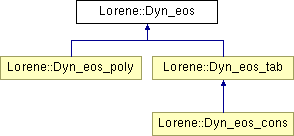List of all members.

## Public Member Functions

virtual ~Dyn_eos ()
Destructor.
const string & get_name () const
Returns the EOS name.
void set_name (const string &)
Sets the EOS name.
virtual bool operator== (const Dyn_eos &) const =0
Comparison operator (egality).
virtual bool operator!= (const Dyn_eos &) const =0
Comparison operator (difference).
virtual int identify () const =0
Returns a number to identify the sub-classe of Dyn_eos the object belongs to.
virtual void sauve (FILE *) const
Save in a file.
virtual double ent_nbar_p (double nbar, const Param *par=0x0) const =0
Computes the log-enthalpy from the baryon density and extra parameters (virtual function implemented in the derived classes).
Scalar ent_nbar (const Scalar &nbar, int nzet, int l_min=0, Param *par=0x0) const
Computes the log-enthalpy field from the baryon density field and extra parameters.
virtual double ener_nbar_p (double nbar, const Param *par=0x0) const =0
Computes the total energy density from the baryon density and extra parameters (virtual function implemented in the derived classes).
Scalar ener_nbar (const Scalar &nbar, int nzet, int l_min=0, Param *par=0x0) const
Computes the total energy density from the baryon density and extra parameters.
virtual double press_nbar_p (double nbar, const Param *par=0x0) const =0
Computes the pressure from the baryon density and extra parameters (virtual function implemented in the derived classes).
Scalar press_nbar (const Scalar &nbar, int nzet, int l_min=0, Param *par=0x0) const
Computes the pressure from the baryon density and extra parameters.
virtual double csound_nbar_p (double nbar, const Param *par=0x0) const =0
Computes the sound speed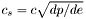from the baryon density with extra parameters (virtual function implemented in the derived classes).
Scalar csound_nbar (const Scalar &nbar, int nzet, int l_min=0, Param *par=0x0) const
Computes the sound speedfrom the baryon density with extra parameters.

## Static Public Member Functions

static Dyn_eoseos_from_file (FILE *)
Construction of an EOS from a binary file.
static Dyn_eoseos_from_file (ifstream &)
Construction of an EOS from a formatted file.

## Protected Member Functions

Dyn_eos ()
Standard constructor.
Dyn_eos (const string &)
Standard constructor with name.
Dyn_eos (const Dyn_eos &)
Copy constructor.
Dyn_eos (FILE *)
Constructor from a binary file (created by the function sauve(FILE*) ).
Dyn_eos (ifstream &)
Constructor from a formatted file.
virtual ostream & operator>> (ostream &) const =0
Operator >>.
void calcule (const Scalar &thermo, int nzet, int l_min, double(Dyn_eos::*fait)(double, const Param *) const, Param *par, Scalar &resu) const
General computational method for Scalar 's.

string name
EOS name.

## Friends

ostream & operator<< (ostream &, const Dyn_eos &)
Display.

## Detailed Description

Equation of state for use in dynamical code base class.

()

This class describes equation of states with baryon density as parameter as opposed to the classes of the group Eos where it is the log-enthalpy which is used.

Definition at line 68 of file dyneos.h.

## Constructor & Destructor Documentation

 Lorene::Dyn_eos::Dyn_eos ( )  [protected]

Standard constructor.

Definition at line 60 of file dyneos.C.

 Lorene::Dyn_eos::Dyn_eos ( const string & name_i )  [explicit, protected]

Standard constructor with name.

Definition at line 64 of file dyneos.C.

 Lorene::Dyn_eos::Dyn_eos ( const Dyn_eos & eos_i )  [protected]

Copy constructor.

Definition at line 68 of file dyneos.C.

 Lorene::Dyn_eos::Dyn_eos ( FILE * fich )  [protected]

Constructor from a binary file (created by the function sauve(FILE*) ).

This constructor is protected because any EOS construction from a binary file must be done via the function Dyn_eos::eos_from_file(FILE*) .

Definition at line 72 of file dyneos.C.

References name.

 Lorene::Dyn_eos::Dyn_eos ( ifstream & fich )  [protected]

Constructor from a formatted file.

This constructor is protected because any EOS construction from a formatted file must be done via the function Dyn_eos::eos_from_file(ifstream&) .

Definition at line 83 of file dyneos.C.

References name.

 Lorene::Dyn_eos::~Dyn_eos ( )  [virtual]

Destructor.

Definition at line 92 of file dyneos.C.

## Member Function Documentation

 void Lorene::Dyn_eos::calcule ( const Scalar & thermo, int nzet, int l_min, double(Dyn_eos::*)(double, const Param *) const fait, Param * par, Scalar & resu ) const [protected]

General computational method for Scalar 's.

Parameters:
 thermo [input] thermodynamical quantity (for instance the density field) from which the thermodynamical quantity resu is to be computed. nzet [input] number of domains where resu is to be computed. l_min [input] index of the innermost domain is which resu is to be computed [default value: 0]; resu is computed only in domains whose indices are in [l_min,l_min+nzet-1] . In the other domains, it is set to zero. fait [input] pointer on the member function of class Dyn_eos which performs the pointwise calculation. par possible extra parameters of the EOS resu [output] result of the computation.

Definition at line 129 of file dyneos.C.

 Scalar Lorene::Dyn_eos::csound_nbar ( const Scalar & nbar, int nzet, int l_min = 0, Param * par = 0x0 ) const

Computes the sound speedfrom the baryon density with extra parameters.

Parameters:
 nbar [input, unit: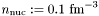] baryon density nzet number of domains where the derivative dln(e)/dln(H) is to be computed. l_min index of the innermost domain is which the coefficient dln(n)/dln(H) is to be computed [default value: 0]; the derivative dln(e)/dln(H) is computed only in domains whose indices are in [l_min,l_min+nzet-1] . In the other domains, it is set to zero. par possible extra parameters of the EOS
Returns: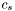[unit: c]

Definition at line 233 of file dyneos.C.

References calcule(), csound_nbar_p(), and Lorene::Tensor::get_mp().

 virtual double Lorene::Dyn_eos::csound_nbar_p ( double nbar, const Param * par = 0x0 ) const [pure virtual]

Computes the sound speedfrom the baryon density with extra parameters (virtual function implemented in the derived classes).

Parameters:
 nbar [input, unit:] baryon density par possible extra parameters of the EOS
Returns:[unit: c]

Implemented in Lorene::Dyn_eos_poly, and Lorene::Dyn_eos_tab.

 Scalar Lorene::Dyn_eos::ener_nbar ( const Scalar & nbar, int nzet, int l_min = 0, Param * par = 0x0 ) const

Computes the total energy density from the baryon density and extra parameters.

Parameters:
 nbar [input, unit:] baryon density nzet number of domains where the energy density is to be computed. l_min index of the innermost domain is which the energy density is to be computed [default value: 0]; the energy density is computed only in domains whose indices are in [l_min,l_min+nzet-1] . In the other domains, it is set to zero. par possible extra parameters of the EOS
Returns:
energy density [unit: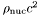], where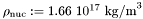Definition at line 209 of file dyneos.C.

References calcule(), ener_nbar_p(), and Lorene::Tensor::get_mp().

 virtual double Lorene::Dyn_eos::ener_nbar_p ( double nbar, const Param * par = 0x0 ) const [pure virtual]

Computes the total energy density from the baryon density and extra parameters (virtual function implemented in the derived classes).

Parameters:
 nbar [input, unit:] baryon density par possible extra parameters of the EOS
Returns:
energy density e [unit:], whereImplemented in Lorene::Dyn_eos_poly, and Lorene::Dyn_eos_tab.

 Scalar Lorene::Dyn_eos::ent_nbar ( const Scalar & nbar, int nzet, int l_min = 0, Param * par = 0x0 ) const

Computes the log-enthalpy field from the baryon density field and extra parameters.

Parameters:
 nbar [input, unit:] baryon density nzet number of domains where the baryon density is to be computed. l_min index of the innermost domain is which the baryon density is to be computed [default value: 0]; the baryon density is computed only in domains whose indices are in [l_min,l_min+nzet-1] . In the other domains, it is set to zero. par possible extra parameters of the EOS
Returns:
ent log-enthalpy H defined by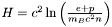, where e is the (total) energy density, p the pressure, n the baryon density, and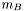the baryon mass.

Definition at line 196 of file dyneos.C.

References calcule(), ent_nbar_p(), and Lorene::Tensor::get_mp().

 virtual double Lorene::Dyn_eos::ent_nbar_p ( double nbar, const Param * par = 0x0 ) const [pure virtual]

Computes the log-enthalpy from the baryon density and extra parameters (virtual function implemented in the derived classes).

Parameters:
 nbar [input, unit:] baryon density par possible extra parameters of the EOS
Returns:
ent log-enthalpy H defined by, where e is the (total) energy density, p the pressure, n the baryon density, andthe baryon mass.

Implemented in Lorene::Dyn_eos_poly, and Lorene::Dyn_eos_tab.

 Dyn_eos * Lorene::Dyn_eos::eos_from_file ( ifstream & fich )  [static]

Construction of an EOS from a formatted file.

The fist line of the file must start by the EOS number, according to the following conventions (same as fo the classes Eos ):

• 1 = relativistic polytropic EOS (class Dyn_eos_poly ).
• 2 = Newtonian polytropic EOS (class Dyn_eos_poly_newt ).
• 17 = Tabulated EOS (class Dyn_eos_tab ).
• 20 = Consistent EOS from table (class Dyn_eos_cons ).

The second line in the file should contain a name given by the user to the EOS. The following lines should contain the EOS parameters (one parameter per line), in the same order than in the class declaration.

Definition at line 295 of file dyneos.C.

 Dyn_eos * Lorene::Dyn_eos::eos_from_file ( FILE * fich )  [static]

Construction of an EOS from a binary file.

The file must have been created by the function sauve(FILE*) .

Definition at line 256 of file dyneos.C.

 const string & Lorene::Dyn_eos::get_name ( ) const

Returns the EOS name.

Definition at line 103 of file dyneos.C.

References name.

 virtual int Lorene::Dyn_eos::identify ( ) const [pure virtual]

Returns a number to identify the sub-classe of Dyn_eos the object belongs to.

Implemented in Lorene::Dyn_eos_poly, Lorene::Dyn_eos_tab, and Lorene::Dyn_eos_cons.

 virtual bool Lorene::Dyn_eos::operator!= ( const Dyn_eos & ) const [pure virtual]

Comparison operator (difference).

 virtual bool Lorene::Dyn_eos::operator== ( const Dyn_eos & ) const [pure virtual]

Comparison operator (egality).

 virtual ostream& Lorene::Dyn_eos::operator>> ( ostream & ) const [protected, pure virtual]
 Scalar Lorene::Dyn_eos::press_nbar ( const Scalar & nbar, int nzet, int l_min = 0, Param * par = 0x0 ) const

Computes the pressure from the baryon density and extra parameters.

Parameters:
 nbar [input, unit:] baryon density nzet number of domains where the pressure is to be computed. l_min index of the innermost domain is which the pressure is to be computed [default value: 0]; the pressure is computed only in domains whose indices are in [l_min,l_min+nzet-1] . In the other domains, it is set to zero. par possible extra parameters of the EOS
Returns:
pressure [unit:], whereDefinition at line 221 of file dyneos.C.

References calcule(), Lorene::Tensor::get_mp(), and press_nbar_p().

 virtual double Lorene::Dyn_eos::press_nbar_p ( double nbar, const Param * par = 0x0 ) const [pure virtual]

Computes the pressure from the baryon density and extra parameters (virtual function implemented in the derived classes).

Parameters:
 nbar [input, unit:] baryon density par possible extra parameters of the EOS
Returns:
pressure p [unit:], whereImplemented in Lorene::Dyn_eos_poly, and Lorene::Dyn_eos_tab.

 void Lorene::Dyn_eos::sauve ( FILE * fich ) const [virtual]

Save in a file.

Reimplemented in Lorene::Dyn_eos_poly, and Lorene::Dyn_eos_tab.

Definition at line 111 of file dyneos.C.

References Lorene::fwrite_be(), identify(), and name.

 void Lorene::Dyn_eos::set_name ( const string & name_i )

Sets the EOS name.

Definition at line 98 of file dyneos.C.

References name.

## Friends And Related Function Documentation

 ostream& operator<< ( ostream & , const Dyn_eos & )  [friend]

Display.

## Member Data Documentation

 string Lorene::Dyn_eos::name [protected]

EOS name.

Definition at line 74 of file dyneos.h.

The documentation for this class was generated from the following files:

Generated on 7 Dec 2019 for LORENE by1.6.1